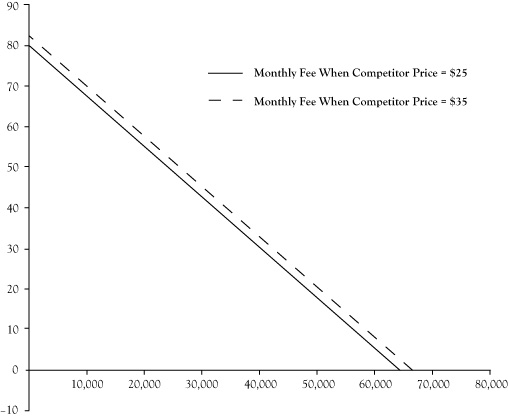# 3.4: Modeling Consumer Demand

•• Anonymous
• LibreTexts
$$\newcommand{\vecs}{\overset { \rightharpoonup} {\mathbf{#1}} }$$ $$\newcommand{\vecd}{\overset{-\!-\!\rightharpoonup}{\vphantom{a}\smash {#1}}}$$$$\newcommand{\id}{\mathrm{id}}$$ $$\newcommand{\Span}{\mathrm{span}}$$ $$\newcommand{\kernel}{\mathrm{null}\,}$$ $$\newcommand{\range}{\mathrm{range}\,}$$ $$\newcommand{\RealPart}{\mathrm{Re}}$$ $$\newcommand{\ImaginaryPart}{\mathrm{Im}}$$ $$\newcommand{\Argument}{\mathrm{Arg}}$$ $$\newcommand{\norm}{\| #1 \|}$$ $$\newcommand{\inner}{\langle #1, #2 \rangle}$$ $$\newcommand{\Span}{\mathrm{span}}$$ $$\newcommand{\id}{\mathrm{id}}$$ $$\newcommand{\Span}{\mathrm{span}}$$ $$\newcommand{\kernel}{\mathrm{null}\,}$$ $$\newcommand{\range}{\mathrm{range}\,}$$ $$\newcommand{\RealPart}{\mathrm{Re}}$$ $$\newcommand{\ImaginaryPart}{\mathrm{Im}}$$ $$\newcommand{\Argument}{\mathrm{Arg}}$$ $$\newcommand{\norm}{\| #1 \|}$$ $$\newcommand{\inner}{\langle #1, #2 \rangle}$$ $$\newcommand{\Span}{\mathrm{span}}$$$$\newcommand{\AA}{\unicode[.8,0]{x212B}}$$

To develop a formal model of consumer demand, the first step is to identify the most important determinants of demand and define variables that measure those determinants. Ideally, we should use variables for which data exist so that statistical estimation techniques can be applied to develop an algebraic relationship between the units of a good consumed and the values of the key determinants. Techniques to derive these algebraic relationships from historical data are outside the scope of this text, but an interested reader may want to consult a text on econometrics. Stock and Watson (2007) is an established econometrics text.

We will examine a simple example of a model of consumer demand. Suppose a business is selling broadband services in a community. The managers of the business have identified four key determinants of demand: (a) the price they charge for the service, (b) their advertising expenditure, (c) the price charged by the competition, and (d) the disposable income of their potential customers. They define four variables to measure these determinants:

• $$P$$ = the price per month of their service, in dollars,
• $$A$$ = advertising expenditure per month, in dollars,
• $$CP$$ = the price per month of the competitor’s service, in dollars,
• $$DIPC$$ = the disposable income per capita, in dollars, as measured by the U.S. Department of Commerce for that month.

Using past data, they estimate the following equation to relate these variables to number of broadband subscribers to their service during a month, symbolized by $$Q$$:

$Q = 25,800 − 800 P + 4 A + 200 CP + 0.4 DIPC.$

This relationship is called a demand function.

One application of the demand function is to estimate the consumption quantity $$Q$$ for specific values of $$P$$, $$CP$$, and $$DIPC$$. Suppose $$P$$ = $30, A =$5000, CP = $25, and $$DIPC$$ =$33,000:

$Q = 25,800 − 800(30) + 4(5000) + 200(25) + 0.4(33,000) = 40,000 \,\text{subscribers}$

In Chapter 2, we introduced a demand curve to describe the relationship between the quantity of items sold and the price of the item. When there are multiple determinants of demand, the demand curve can be interpreted as a reduced view of the demand function where only the price of the product is allowed to vary. Any other variables are assumed to remain at a fixed level. For the previous demand function for broadband service, suppose we assume A is fixed at $5000, $$CP$$ is fixed at$25, and $$DIPC$$ is fixed at $33,000. If you substitute these values into the demand function and aggregate constant terms, the demand function becomes $Q = 64,000 − 800 P.$ Recall that demand curves are usually expressed with price as a function of quantity. With some basic algebra the equation of the demand curve can be written as $P = 80 − 0.00125 Q.$ What happens to the demand curve if one of the other variables is a different value? Well, in short, the demand curve would shift. Suppose the competitor decides to increase its price to$35. Repeating the preceding steps, the demand function simplifies to

$Q = 66,000 − 800 P$

or, expressed with $$P$$ as a function of Q,

$P = 82.50 − 0.00125 Q.$

Figure 3.1 shows a graph of the demand curve before and after the shift. Effectively, the result is that the broadband firm would see its demand increase by 2000 customers per month, or alternatively, the firm could raise its price to $32.50 and still maintain 40,000 customers per month.Figure 3.1 Shift in Demand Curve for Broadband Service Caused by Increase of Competitor Price From$25/month to \$35/month

This page titled 3.4: Modeling Consumer Demand is shared under a CC BY-NC-SA 3.0 license and was authored, remixed, and/or curated by Anonymous via source content that was edited to the style and standards of the LibreTexts platform; a detailed edit history is available upon request.